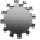Plenary Lecture

Generalized Least-Squares RegressionProfessor Nataniel Greene
Department of Mathematics and Computer Science
Kingsborough Community College
City University of New York
USA
E-mail: ngreene.math@gmail.com

Abstract: Ordinary least-squares regression suffers from a fundamental lack of symmetry: the inverse of the regression line of y given x is not the regression line of x given y. Alternative symmetric regression methods have been developed to address this concern, notably: orthogonal regression and geometric mean regression. In our previous work a variety of symmetric and weighted least-squares regression methods were derived and analyzed, some of which may not have been known or fully explicated. A general approach to deriving, analyzing and classifying all generalized least-squares methods was also developed. This talk describes the further development of this theory.

Brief Biography of the Speaker: TBA